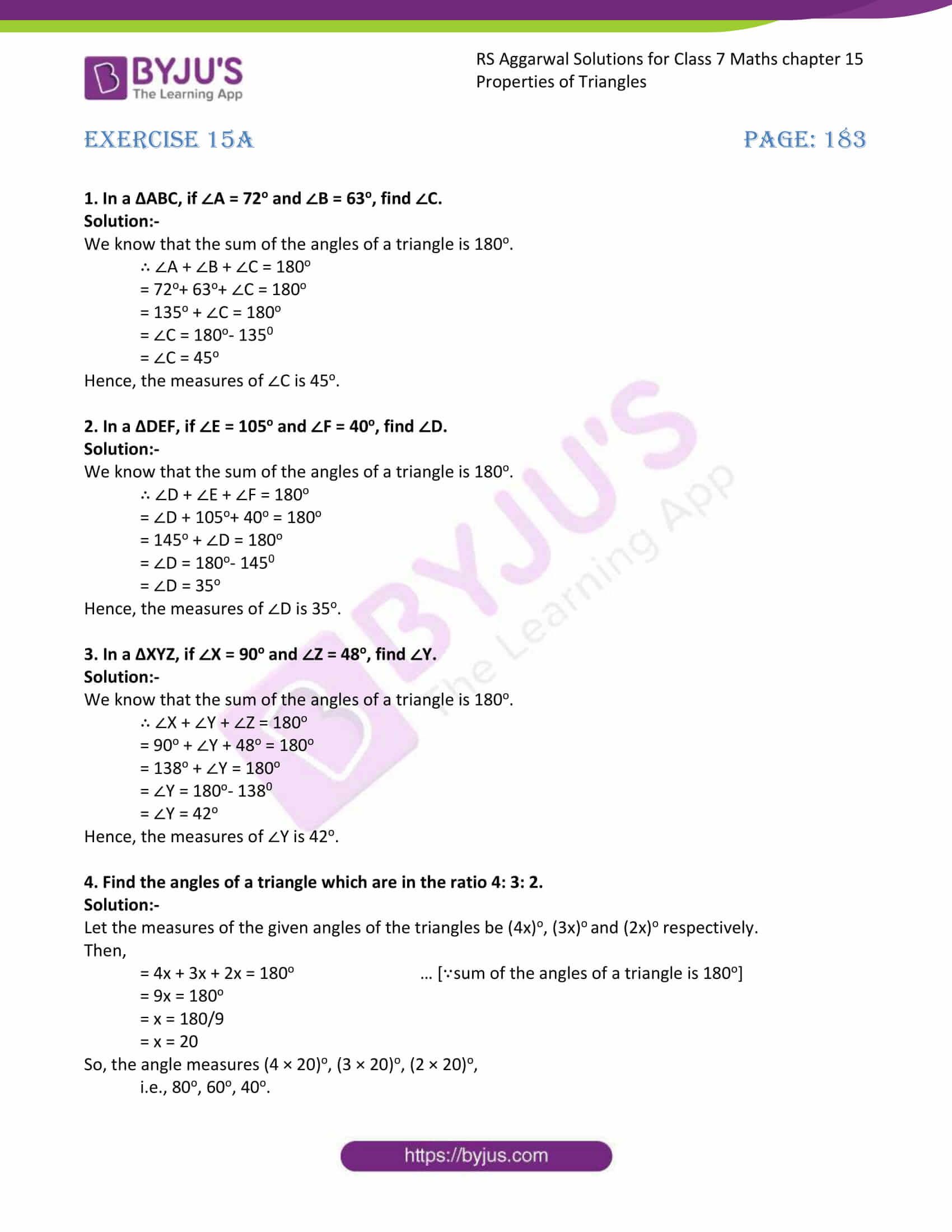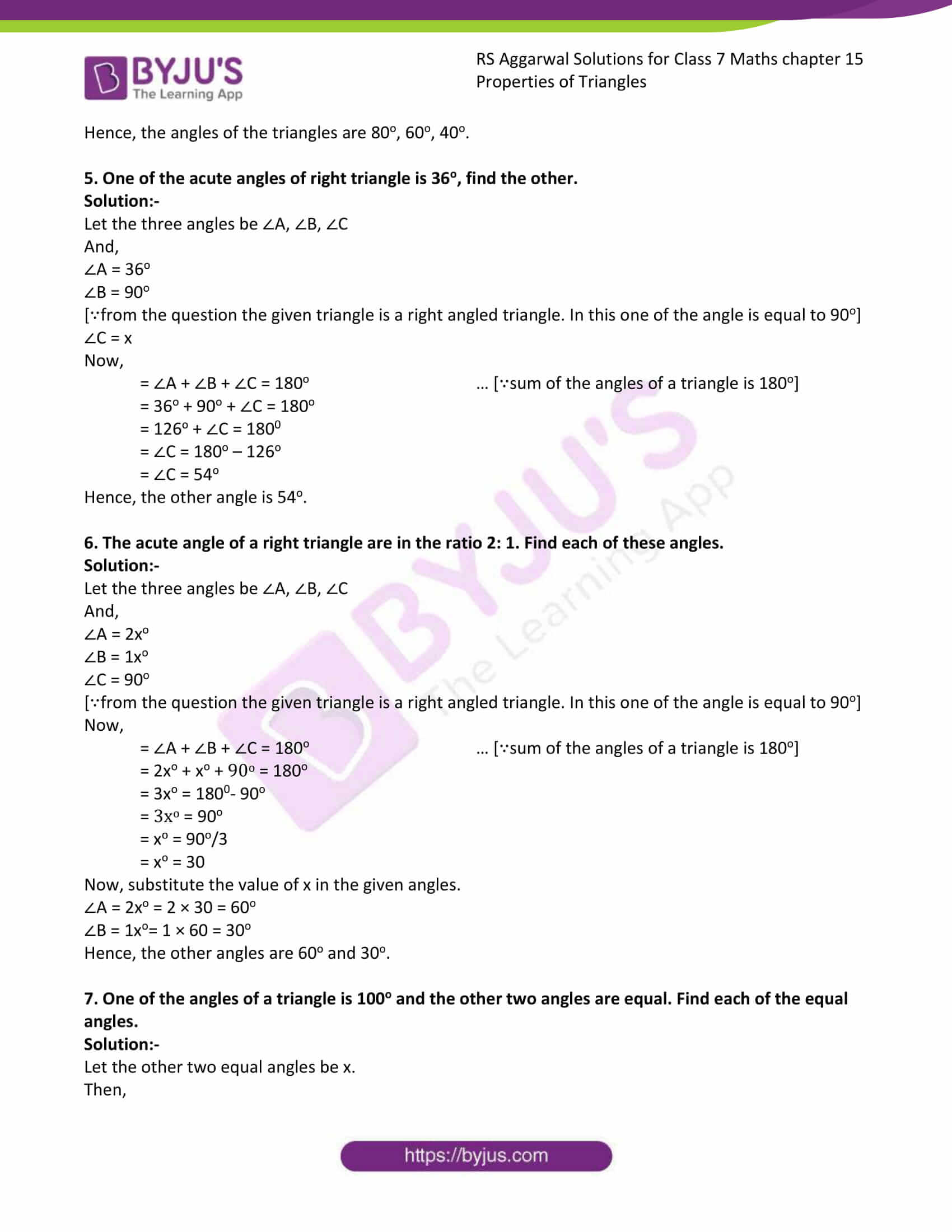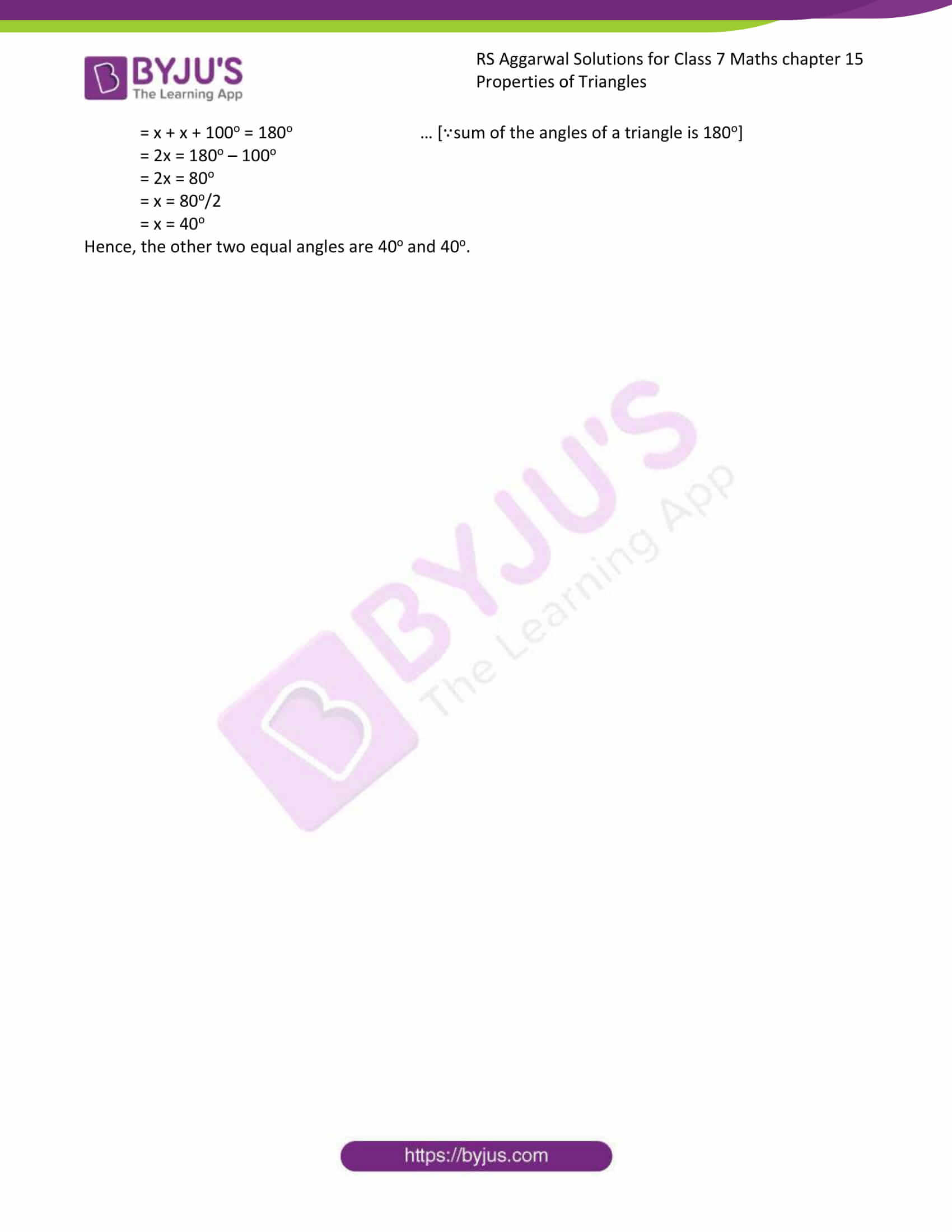# RS Aggarwal Solutions for Class 7 Maths Exercise 15A Chapter 15 Properties of Triangles

RS Aggarwal Solutions for Class 7 Maths Exercise 15A Chapter 15 Properties of Triangles, Download PDF are given here. This exercise of RS Aggarwal Solutions for Class 7 Maths Chapter 15 contains questions related to various types of triangles and angle sum property of a triangle. We at Byju’s have prepared the solutions with stepwise containing neat descriptions. Students can score more marks in Maths by practising RS Aggarwal Solutions for Class 7 Maths Chapter 15 Properties of Triangles.

## Download the PDF of RS Aggarwal Solutions For Class 7 Maths Chapter 15 Properties of Triangles – Exercise 15A### Access answers to Maths RS Aggarwal Solutions for Class 7 Chapter 15 – Properties of Triangles Exercise 15A

1. In a ΔABC, if ∠A = 72o and ∠B = 63o, find ∠C.

Solution:-

We know that the sum of the angles of a triangle is 180o.

∴ ∠A + ∠B + ∠C = 180o

= 72o+ 63o+ ∠C = 180o

= 135o + ∠C = 180o

= ∠C = 180o– 1350

= ∠C = 45o

Hence, the measures of ∠C is 45o.

2. In a ΔDEF, if ∠E = 105o and ∠F = 40o, find ∠D.

Solution:-

We know that the sum of the angles of a triangle is 180o.

∴ ∠D + ∠E + ∠F = 180o

= ∠D + 105o+ 40o = 180o

= 145o + ∠D = 180o

= ∠D = 180o– 1450

= ∠D = 35o

Hence, the measures of ∠D is 35o.

3. In a ΔXYZ, if ∠X = 90o and ∠Z = 48o, find ∠Y.

Solution:-

We know that the sum of the angles of a triangle is 180o.

∴ ∠X + ∠Y + ∠Z = 180o

= 90o + ∠Y + 48o = 180o

= 138o + ∠Y = 180o

= ∠Y = 180o– 1380

= ∠Y = 42o

Hence, the measures of ∠Y is 42o.

4. Find the angles of a triangle which are in the ratio 4: 3: 2.

Solution:-

Let the measures of the given angles of the triangles be (4x)o, (3x)o and (2x)o respectively.

Then,

= 4x + 3x + 2x = 180o … [∵sum of the angles of a triangle is 180o]

= 9x = 180o

= x = 180/9

= x = 20

So, the angle measures (4 × 20)o, (3 × 20)o, (2 × 20)o,

i.e., 80o, 60o, 40o.

Hence, the angles of the triangles are 80o, 60o, 40o.

5. One of the acute angles of right triangle is 36o, find the other.

Solution:-

Let the three angles be ∠A, ∠B, ∠C

And,

∠A = 36o

∠B = 90o

[∵from the question the given triangle is a right angled triangle. In this one of the angle is equal to 90o]

∠C = x

Now,

= ∠A + ∠B + ∠C = 180o … [∵sum of the angles of a triangle is 180o]

= 36o + 90o + ∠C = 180o

= 126o + ∠C = 1800

= ∠C = 180o – 126o

= ∠C = 54o

Hence, the other angle is 54o.

6. The acute angle of a right triangle are in the ratio 2: 1. Find each of these angles.

Solution:-

Let the three angles be ∠A, ∠B, ∠C

And,

∠A = 2xo

∠B = 1xo

∠C = 90o

[∵from the question the given triangle is a right angled triangle. In this one of the angle is equal to 90o]

Now,

= ∠A + ∠B + ∠C = 180o … [∵sum of the angles of a triangle is 180o]

= 2xo + xo + 90o = 180o

= 3xo = 1800– 90o

= 3xo = 90o

= xo = 90o/3

= xo = 30

Now, substitute the value of x in the given angles.

∠A = 2xo = 2 × 30 = 60o

∠B = 1xo= 1 × 60 = 30o

Hence, the other angles are 60o and 30o.

7. One of the angles of a triangle is 100o and the other two angles are equal. Find each of the equal angles.

Solution:-

Let the other two equal angles be x.

Then,

= x + x + 100o = 180o … [∵sum of the angles of a triangle is 180o]

= 2x = 180o – 100o

= 2x = 80o

= x = 80o/2

= x = 40o

Hence, the other two equal angles are 40o and 40o.

### Access other exercises of RS Aggarwal Solutions For Class 7 Chapter 15 – Properties of Triangles

Exercise 15B Solutions

Exercise 15C Solutions

Exercise 15D Solution